Inductor AC Response

 Impedance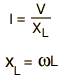CalculateExamine Capacitor ResistorContribution tocomplex impedance Phasor diagram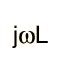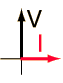You know that the voltage across an inductor leads the current because the Lenz' law behavior resists the buildup of the current, and it takes a finite time for an imposed voltage to force the buildup of current to its maximum.

Index

Capacitance concepts

Inductance concepts

AC Circuits

 HyperPhysics***** Electricity and Magnetism R Nave
Go Back

Inductive Reactance

The frequency dependent impedance of an inductor is called inductive reactance.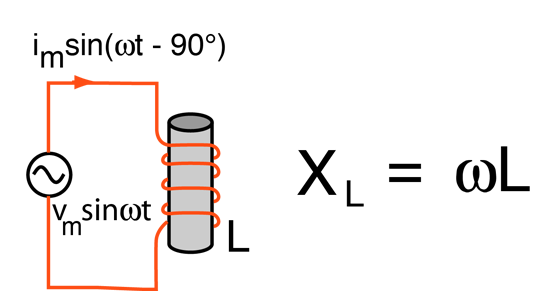This calculation works by clicking on the desired quantity in the expression below. Enter the necessary data and then click on the quantity you wish to calculate. Default values will be entered for unspecified quantities, but all quantities may be changed.

Impedance = Angular frequency x Inductance

 For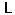= x10^ H = mH = microHenries
 at angular frequency ω = x10^ rad/s
and frequency = x10^ Hz = kHz = MHz

the inductive reactance is

 XL = x10^ ohms = kohms = Megohms
 AC behavior of inductor
Index

Capacitance concepts

Inductance concepts

AC Circuits

 HyperPhysics***** Electricity and Magnetism R Nave
Go Back